Like   Tweet
 /* styles */ April 15, 2020 Dear Readers, This is our second installment of “Expand Your Horizons While Sheltering at Home.” We would like to reintroduce you to the 2018 Finalists for the Dayton Literary Peace Prize who come from throughout the world—their countries of origin include the Czech Republic, Germany, Great Britain, South Korea, Palestine, Pakistan, Taiwan, and the U.S. Their books explore issues of immigration, occupation, ethnic wars, the power of education, justice and injustice, and African American history. We can learn much from their books and gain perspective on the current state of affairs at home and abroad, realizing that like the movement of the famed butterfly’s wing, the plight of people seemingly a world away has an effect that echoes throughout the globe. We invite you to meet the characters and people in these books whose lives connect with ours. Stay safe,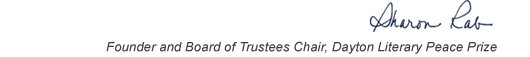table div table+table+table+table div table{width:100%;padding:0}table div table+table+table+table div table img{width:96.23%;padding:0;float:none}table div table+table+table+table div table td{width:100%;padding:0 1.88% 18px}/* styles */This list includes the winners and runners-up for 2018 and we have rich background information on each one on our website.

▪ Under Past Winners. scroll down to the year and click on it.
▪ You will have the option of clicking on a winner, a runner-up, or the finalists.
▪ If you click on the photo of winners and runners-up, you will find the author’s photo and bio, a selection from the book, the judges’ citation, the writer’s reflection on literature and peace, and the option of watching the introduction and acceptance speech.
 ▪ Under Past Winners. scroll down to the year and click on it.
 ▪ You will have the option of clicking on a winner, a runner-up, or the finalists.
 ▪ If you click on the photo of winners and runners-up, you will find the author’s photo and bio, a selection from the book, the judges’ citation, the writer’s reflection on literature and peace, and the option of watching the introduction and acceptance speech.

On the bottom right of the home page, you can click on COREScholar, which is built by the Chair of our Curriculum Committee Carol Loranger, Associate Dean of the College of Liberal Arts at Wright State, and maintained for us by Wright State University. There you can find interviews, TED Talks, articles, other books, films, etc. by and about each of our winning and runner-up authors. It is a great resource for students, book clubs, and readers who would like to explore an author in depth.

 table div table+table+table+table+table+table div table{width:100%;padding:0}table div table+table+table+table+table+table div table img{width:96.23%;padding:0;float:none}table div table+table+table+table+table+table div table td{width:100%;padding:0 1.88% 18px}/* styles *//* styles */ “Read global; buy local.” — Marlon James, DLPP 2009 Fiction Winner for The Book of Night Women
 table div table+table+table+table+table+table+table+table div table{width:100%;padding:0}table div table+table+table+table+table+table+table+table div table img{width:96.23%;padding:0;float:none}table div table+table+table+table+table+table+table+table div table td{width:100%;padding:0 1.88% 18px}/* styles */# 2018 Finalists - Fiction

 table div table+table+table+table+table+table+table+table+table+table div table{width:100%;padding:0}table div table+table+table+table+table+table+table+table+table+table div table img{width:96.23%;padding:0;float:none}table div table+table+table+table+table+table+table+table+table+table div table td{width:100%;padding:0 1.88% 18px}/* styles */Exit West by Mohsin Hamid (Riverhead): An astonishingly timely love story that brilliantly imagines the forces that transform ordinary people into refugees and the impossible choices that follow.

 table div table+table+table+table+table+table+table+table+table+table+table+table div table{width:100%;padding:0}table div table+table+table+table+table+table+table+table+table+table+table+table div table img{width:96.23%;padding:0;float:none}table div table+table+table+table+table+table+table+table+table+table+table+table div table td{width:100%;padding:0 1.88% 18px}/* styles */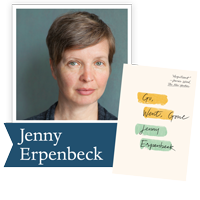Go, Went, Gone by Jenny Erpenbeck (New Directions): A scathing indictment of Western policy toward the European refugee crisis, but also a touching portrait of a man who finds he has more in common with the Africans than he realizes.

 table div table+table+table+table+table+table+table+table+table+table+table+table+table+table div table{width:100%;padding:0}table div table+table+table+table+table+table+table+table+table+table+table+table+table+table div table img{width:96.23%;padding:0;float:none}table div table+table+table+table+table+table+table+table+table+table+table+table+table+table div table td{width:100%;padding:0 1.88% 18px}/* styles */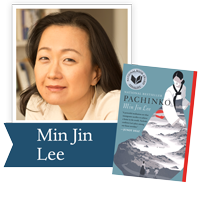Pachinko by Min Jin Lee (Grand Central): Four generations of a poor, proud immigrant Korean family fight to control their destinies, exiled from a homeland they never knew.

 table div table+table+table+table+table+table+table+table+table+table+table+table+table+table+table+table div table{width:100%;padding:0}table div table+table+table+table+table+table+table+table+table+table+table+table+table+table+table+table div table img{width:96.23%;padding:0;float:none}table div table+table+table+table+table+table+table+table+table+table+table+table+table+table+table+table div table td{width:100%;padding:0 1.88% 18px}/* styles */Salt Houses by Hala Alyan (NMH): A heartbreaking story that follows three generations of a Palestinian family and asks us to confront that most devastating of all truths: you can’t go home again.

 table div table+table+table+table+table+table+table+table+table+table+table+table+table+table+table+table+table+table div table{width:100%;padding:0}table div table+table+table+table+table+table+table+table+table+table+table+table+table+table+table+table+table+table div table img{width:96.23%;padding:0;float:none}table div table+table+table+table+table+table+table+table+table+table+table+table+table+table+table+table+table+table div table td{width:100%;padding:0 1.88% 18px}/* styles */Sing, Unburied, Sing by Jesmyn Ward (Scribner): Following a family making the trip from their Gulf Coast town to the Mississippi State Penitentiary, testing the strength of emotional bonds and the pull of our collective history.

 table div table+table+table+table+table+table+table+table+table+table+table+table+table+table+table+table+table+table+table+table div table{width:100%;padding:0}table div table+table+table+table+table+table+table+table+table+table+table+table+table+table+table+table+table+table+table+table div table img{width:96.23%;padding:0;float:none}table div table+table+table+table+table+table+table+table+table+table+table+table+table+table+table+table+table+table+table+table div table td{width:100%;padding:0 1.88% 18px}/* styles */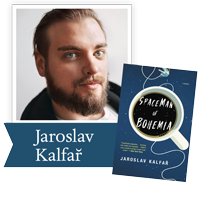Spaceman of Bohemia by Jaroslav Kalfař (Little, Brown): Raised in the Czech countryside by his doting grandparents, Jakub Procházka has risen from small-time scientist to become the country's first astronaut. A dangerous solo mission to Venus offers him the chance at heroism he's dreamt of and a way to atone for his father's sins as a Communist informer.

 table div table+table+table+table+table+table+table+table+table+table+table+table+table+table+table+table+table+table+table+table+table+table div table{width:100%;padding:0}table div table+table+table+table+table+table+table+table+table+table+table+table+table+table+table+table+table+table+table+table+table+table div table img{width:96.23%;padding:0;float:none}table div table+table+table+table+table+table+table+table+table+table+table+table+table+table+table+table+table+table+table+table+table+table div table td{width:100%;padding:0 1.88% 18px}/* styles */# 2018 Finalists - Nonfiction

 table div table+table+table+table+table+table+table+table+table+table+table+table+table+table+table+table+table+table+table+table+table+table+table+table div table{width:100%;padding:0}table div table+table+table+table+table+table+table+table+table+table+table+table+table+table+table+table+table+table+table+table+table+table+table+table div table img{width:96.23%;padding:0;float:none}table div table+table+table+table+table+table+table+table+table+table+table+table+table+table+table+table+table+table+table+table+table+table+table+table div table td{width:100%;padding:0 1.88% 18px}/* styles */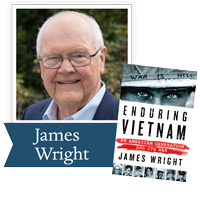Enduring Vietnam by James Wright (St. Martin’s Press): Recounts the experiences of the young Americans who fought in Vietnam and of families who grieved those who did not return.

 table div table+table+table+table+table+table+table+table+table+table+table+table+table+table+table+table+table+table+table+table+table+table+table+table+table+table div table{width:100%;padding:0}table div table+table+table+table+table+table+table+table+table+table+table+table+table+table+table+table+table+table+table+table+table+table+table+table+table+table div table img{width:96.23%;padding:0;float:none}table div table+table+table+table+table+table+table+table+table+table+table+table+table+table+table+table+table+table+table+table+table+table+table+table+table+table div table td{width:100%;padding:0 1.88% 18px}/* styles */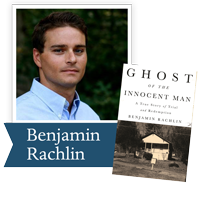Ghost of the Innocent Man by Benjamin Rachlin (Little, Brown): A gripping account of one man's long road to freedom that will forever change how we understand our criminal justice system through one of the most dramatic of those cases. It provides a picture of wrongful conviction and of the opportunity for meaningful reform.

 table div table+table+table+table+table+table+table+table+table+table+table+table+table+table+table+table+table+table+table+table+table+table+table+table+table+table+table+table div table{width:100%;padding:0}table div table+table+table+table+table+table+table+table+table+table+table+table+table+table+table+table+table+table+table+table+table+table+table+table+table+table+table+table div table img{width:96.23%;padding:0;float:none}table div table+table+table+table+table+table+table+table+table+table+table+table+table+table+table+table+table+table+table+table+table+table+table+table+table+table+table+table div table td{width:100%;padding:0 1.88% 18px}/* styles */Lolas’ House by M. Evelina Galang (Northwestern U. Press): Tells the stories, in unprecedented detail, of sixteen surviving Filipino “comfort women.” Not only a book of testimony and documentation, it is a book of witness, of survival, and of the female body.

 table div table+table+table+table+table+table+table+table+table+table+table+table+table+table+table+table+table+table+table+table+table+table+table+table+table+table+table+table+table+table div table{width:100%;padding:0}table div table+table+table+table+table+table+table+table+table+table+table+table+table+table+table+table+table+table+table+table+table+table+table+table+table+table+table+table+table+table div table img{width:96.23%;padding:0;float:none}table div table+table+table+table+table+table+table+table+table+table+table+table+table+table+table+table+table+table+table+table+table+table+table+table+table+table+table+table+table+table div table td{width:100%;padding:0 1.88% 18px}/* styles */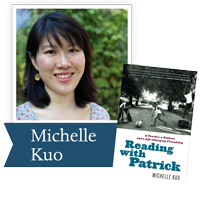Reading with Patrick by Michelle Kuo (Random House): In this stirring memoir, Kuo, the child of Taiwanese immigrants, shares the story of her complicated but rewarding mentorship of one student, Patrick Browning, and his remarkable literary and personal awakening.

 table div table+table+table+table+table+table+table+table+table+table+table+table+table+table+table+table+table+table+table+table+table+table+table+table+table+table+table+table+table+table+table+table div table{width:100%;padding:0}table div table+table+table+table+table+table+table+table+table+table+table+table+table+table+table+table+table+table+table+table+table+table+table+table+table+table+table+table+table+table+table+table div table img{width:96.23%;padding:0;float:none}table div table+table+table+table+table+table+table+table+table+table+table+table+table+table+table+table+table+table+table+table+table+table+table+table+table+table+table+table+table+table+table+table div table td{width:100%;padding:0 1.88% 18px}/* styles */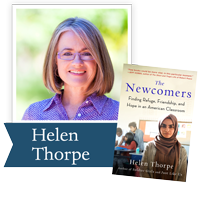The Newcomers by Helen Thorpe (Scribner): Helen Thorpe’s intensive, year-long reporting puts a human face on the faces of 22 newly-arrived teenagers taking a beginner-level English Language Acquisition class at South High School in Denver.

 table div table+table+table+table+table+table+table+table+table+table+table+table+table+table+table+table+table+table+table+table+table+table+table+table+table+table+table+table+table+table+table+table+table+table div table{width:100%;padding:0}table div table+table+table+table+table+table+table+table+table+table+table+table+table+table+table+table+table+table+table+table+table+table+table+table+table+table+table+table+table+table+table+table+table+table div table img{width:96.23%;padding:0;float:none}table div table+table+table+table+table+table+table+table+table+table+table+table+table+table+table+table+table+table+table+table+table+table+table+table+table+table+table+table+table+table+table+table+table+table div table td{width:100%;padding:0 1.88% 18px}/* styles */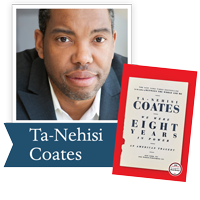We Were Eight Years in Power by Ta-Nehisi Coates (One World PRH): “Biting cultural and political analysis . . . reflects on race, Barack Obama’s presidency and its jarring aftermath, and his evolution as a writer in eight stunningly incisive essays. Kirkus Reviews (starred review)

 table div table+table+table+table+table+table+table+table+table+table+table+table+table+table+table+table+table+table+table+table+table+table+table+table+table+table+table+table+table+table+table+table+table+table+table+table div table{width:100%;padding:0}table div table+table+table+table+table+table+table+table+table+table+table+table+table+table+table+table+table+table+table+table+table+table+table+table+table+table+table+table+table+table+table+table+table+table+table+table div table img{width:96.23%;padding:0;float:none}table div table+table+table+table+table+table+table+table+table+table+table+table+table+table+table+table+table+table+table+table+table+table+table+table+table+table+table+table+table+table+table+table+table+table+table+table div table td{width:100%;padding:0 1.88% 18px}/* styles */## 2018 Ambassador Richard C. Holbrooke Distinguished Achievement Award WinnerThe New York Times 1989 book review of John Irving's A Prayer for Owen Meany:

Our Presidents continue to pour the soothing syrup. But some of our most talented novelists see the political condition of American society as a disaster, the temper of many Americans as correspondingly dangerous. In "A Prayer for Owen Meany" John Irving makes it all too plain, and with positive rage, that in his eyes American society has been a moral disaster since the 1960's. He instances the America that snickered at President Kennedy's amours in the White House, the Vietnam War that sacrificed more than 58,000 of our young men, the moralizing and piety of national leaders who refuse to hinder the traffic in weapons of every kind, to say nothing of a widespread appetite for drugs and the "junk food" of television, which "gives good disaster." Read more.

 table div table+table+table+table+table+table+table+table+table+table+table+table+table+table+table+table+table+table+table+table+table+table+table+table+table+table+table+table+table+table+table+table+table+table+table+table+table+table+table div table{width:100%;padding:0}table div table+table+table+table+table+table+table+table+table+table+table+table+table+table+table+table+table+table+table+table+table+table+table+table+table+table+table+table+table+table+table+table+table+table+table+table+table+table+table div table img{width:96.23%;padding:0;float:none}table div table+table+table+table+table+table+table+table+table+table+table+table+table+table+table+table+table+table+table+table+table+table+table+table+table+table+table+table+table+table+table+table+table+table+table+table+table+table+table div table td{width:100%;padding:0 1.88% 18px}/* styles */table div table+table+table+table+table+table+table+table+table+table+table+table+table+table+table+table+table+table+table+table+table+table+table+table+table+table+table+table+table+table+table+table+table+table+table+table+table+table+table+table+table div table{width:100%;padding:0}table div table+table+table+table+table+table+table+table+table+table+table+table+table+table+table+table+table+table+table+table+table+table+table+table+table+table+table+table+table+table+table+table+table+table+table+table+table+table+table+table+table div table img{width:96.23%;padding:0;float:none}table div table+table+table+table+table+table+table+table+table+table+table+table+table+table+table+table+table+table+table+table+table+table+table+table+table+table+table+table+table+table+table+table+table+table+table+table+table+table+table+table+table div table td{width:100%;padding:0 1.88% 18px}/* styles */“Plague stories remind us that we cannot manage without community . . . Year of Wonders is a testament to that very notion.” – The Washington Post

An unforgettable tale, set in 17th century England, of a village that quarantines itself to arrest the spread of the plague, from the author of The Secret Chord and of March, winner of the Pulitzer Prize.

Ron Rollins, Dayton Daily News Ideas and Voices Editor, will moderate the discussion of The Year of Wonders.

Email Emily Kretzer to be added to the list. We'll send details as they become available.

 table div table+table+table+table+table+table+table+table+table+table+table+table+table+table+table+table+table+table+table+table+table+table+table+table+table+table+table+table+table+table+table+table+table+table+table+table+table+table+table+table+table+table+table div table{width:100%;padding:0}table div table+table+table+table+table+table+table+table+table+table+table+table+table+table+table+table+table+table+table+table+table+table+table+table+table+table+table+table+table+table+table+table+table+table+table+table+table+table+table+table+table+table+table div table img{width:96.23%;padding:0;float:none}table div table+table+table+table+table+table+table+table+table+table+table+table+table+table+table+table+table+table+table+table+table+table+table+table+table+table+table+table+table+table+table+table+table+table+table+table+table+table+table+table+table+table+table div table td{width:100%;padding:0 1.88% 18px}/* styles */Support the Dayton Literary Peace Prize when you shop with Amazon using this link.